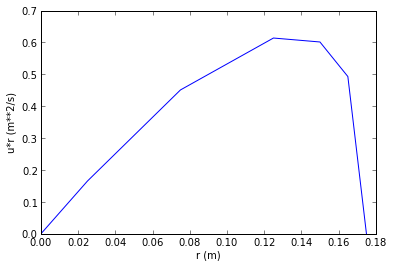# Chapter 4 : Flow Measurement¶

### example 4.1 page no : 65¶

In :
import math

# Initialization of Variable
rho=998.
rhom=1.354*10**4#density  of mercury
M=2.83/100
mu=1.001/1000
mun=1.182/10**5#vicosity of natural gas
R=8.314
g=9.81
h=28.6/100
d=54./100

#part1
nu=1./rho
delP=h*g*(rhom-rho)
umax=math.sqrt(2.*nu*delP)
umax=round(umax*10.)/10
print "maximum fluid velocity in (m/s)",umax
Re=umax*d*rho/mu
print "reynold no. is %.2e"%(Re)

#using chart
u=0.81*umax
G=rho*math.pi*d**2./4*u
print "mass flow rate in (kg/s): %.4f"%G
print "Volumetric flow rate in (m**3/s): %.4f"%(G/rho)

#part2
P1=689.*1000            #initial pressure
T=273+21.
nu1=R*T/M/P1
nu1=round(nu1*10000)/10000.
rhog=1./nu1              #density of gas
h=17.4/100
P2=P1+h*(rho-rhog)*g
P2=round(P2/100)*100.
umax2=math.sqrt(2*P1*nu1*math.log(P2/P1))
print "maximum fluid velocity in (m/s) %.4f"%umax2
Re=rhog*umax2*d/mun
print "reynold no. is %.3e"%(Re)
#from table
u=0.81*umax2
Q=math.pi*d**2/4*u
print "volumetric flow rate is (m**3/s): %.4f"%Q
print "mass flow rate  in (kg/s): %.4f"%(Q*rhog)

maximum fluid velocity in (m/s) 8.4
reynold no. is 4.52e+06
mass flow rate in (kg/s): 1555.1499
Volumetric flow rate in (m**3/s): 1.5583
maximum fluid velocity in (m/s) 20.6358
reynold no. is 7.518e+06
volumetric flow rate is (m**3/s): 3.8281
mass flow rate  in (kg/s): 30.5271


### example 4.2 page no : 67¶

In :
import math
import numpy
from matplotlib import pyplot as plt
%matplotlib inline

# Initialization of Variable
rd = numpy.true_divide([0, 1, 2.5, 5 ,10, 15, 17.5],100)          #radial distance from pipe
dlv = numpy.true_divide([0,0.2, 0.36, 0.54, 0.81, 0.98, 1],100)          #differnce in liquid levels
r = [.175 ,.165, .150, .125 ,.075, .025, 0]
g = 9.81
R = 8.314
rho = 999.
temp = 289.
P1 = 148 * 1000.
M = 7.09 / 100.
pi = 3.12
rhoCl2 = P1 * M / R / temp              #density of Cl2
nuCl2 = 1 / rhoCl2                  #specific volume of Cl2
def P2(x):
return P1+x*(rho-rhoCl2)*g

u = [0,0,0,0,0,0,0]
a = [0,0,0,0,0,0,0]

for i in range(7):
y = P2(dlv[i])
u[i] = math.sqrt(2.*P1*nuCl2*math.log(y/P1))
a[i] = u[i] * r[i]

plt.plot(r,a)
plt.xlabel("r (m)")
plt.ylabel("u*r (m**2/s)")
s=0
for i in range(6):          #itegration of the plotted graph
s=abs((r[i]-r[i+1])*.5*(a[i]+a))+s

s=s-0.01
Q=2*pi*s
plt.show()
#Result
print "volumetric flow rate (m**3/s):",Q
print "mass flow rate of chlorine gas (kg/s)",Q*rhoCl2

Populating the interactive namespace from numpy and matplotlib

WARNING: pylab import has clobbered these variables: ['pi']
%pylab --no-import-all prevents importing * from pylab and numpyvolumetric flow rate (m**3/s): 0.455983319661
mass flow rate of chlorine gas (kg/s) 1.99135662691


### example 4.3 page no : 70¶

In :
import math

# Initialization of Variable
pi = 3.14
Cd = 0.61
rho = 999.
rhoo = 877.            #density of oil
g = 9.81
h = 75/100.
d = 12.4/100.         #dia of orifice
d1 = 15/100.          #inside diameter
nuo = 1/rhoo          #specific volume of oil

#calculation
#part1
delP = h*(rho-rhoo)*g
A = pi*d**2./4
G = Cd*A/nuo*math.sqrt(2*nuo*delP/(1-(d/d1)**4))
print "mass flow rate in (kg/s) %.4f"%G

#part2
h = (1.+0.5)*d1
delP = rhoo/2*(G*nuo/Cd/A)**2*(1-(d/d1)**4)+h*rhoo*g
print "pressuer differnce between tapping points %.4f"%delP
delh = (delP-h*rhoo*g)/(rho-rhoo)/g
print "difference in water levels in manometer i (cm) %.4f"%delh

mass flow rate in (kg/s) 12.6544
pressuer differnce between tapping points 2833.3733
difference in water levels in manometer i (cm) 0.7500


### example 4.4 page no : 72¶

In :
import math

# Initialization of Variable
rhom = 1.356*10**4              #density mercury
rhon = 1266.                    #density NaOH
Cd = 0.61
g = 9.81
Cdv = 0.98                      #coeff. of discharge of venturimeter
Cdo = Cd                        #coeff. of discharge of orificemeter
d = 6.5/100
pi = 3.14
A = pi*d**2/4.
Q = 16.5/1000.

#calculation
#part1
delP = g*h*(rhom-rhon)
G = rhon*Q
nun = 1./rhon#specific volume of NaOH
Ao = G*nun/Cd*math.sqrt(1./(2*nun*delP+(G*nun/Cd/A)**2))        #area of orifice
d0 = math.sqrt(4.*Ao/pi)
print "diameter of orifice in (cm): %.4f"%(d0*100)

#part2
a = (Cdv/Cdo)**2
print "ratio of pressure drop  %.4f"%a

diameter of orifice in (cm): 5.8041
ratio of pressure drop  2.5810


### example 4.5 page no : 74¶

In :
import math

# Initialization of Variable
M = 3.995/100
g = 9.81
R = 8.314
Cd = 0.94
temp = 289.
df = 9.5/1000           #diameter of float
Af = math.pi*df**2/4.         #area of float
P = 115.*10.**3.
V = 0.92/10**6
rhoc = 3778.            #density of ceramic

#calculation
rho = P*M/R/temp
nu = 1/rho
P = V*(rhoc-rho)*g/Af
print  "pressure drop over the float in (Pa): %.4f"%P

#part2
x = .15/25.*(25-7.6)
L = df*100.+2*x
L = L/100.
A1 = math.pi*L**2./4
A0 = A1-Af
G = Cd*A0*math.sqrt(2.*rho*P/(1-(A0/A1)**2))
print "mass flow rate in kg/s) is %.3e"%(G)
Q = G/rho
print "Volumetric flow rate in (m**3/s): %f"%Q

pressure drop over the float in (Pa): 480.7971
mass flow rate in kg/s) is 1.475e-03
Volumetric flow rate in (m**3/s): 0.000772


### example 4.6 page no : 77¶

In :
import math

# Initialization of Variable
rho = 999.
rhos = 8020.            #density of steel
g = 9.81
math.pi = 3.14
df = 14.2/1000          #dia of float
Af = math.pi*df**2/4.        #area of float
Cd = 0.97
nu = 1./rho
Q = 4./1000./60
G = Q*rho

#calculation
x = 0.5*(18.8-df*1000)/280*(280-70)
L = df*1000.+2*x
L = L/1000.
A1 = math.pi*L**2./4
A0 = A1-Af
Vf = Af/g/(rhos-rho)/2/nu*(G*nu/Cd/A0)**2*(1-(A0/A1)**2)
m = Vf*rhos
print "mass of float equired in (g): %.4f"%(m*1000)

mass of float equired in (g): 5.1176

In [ ]: